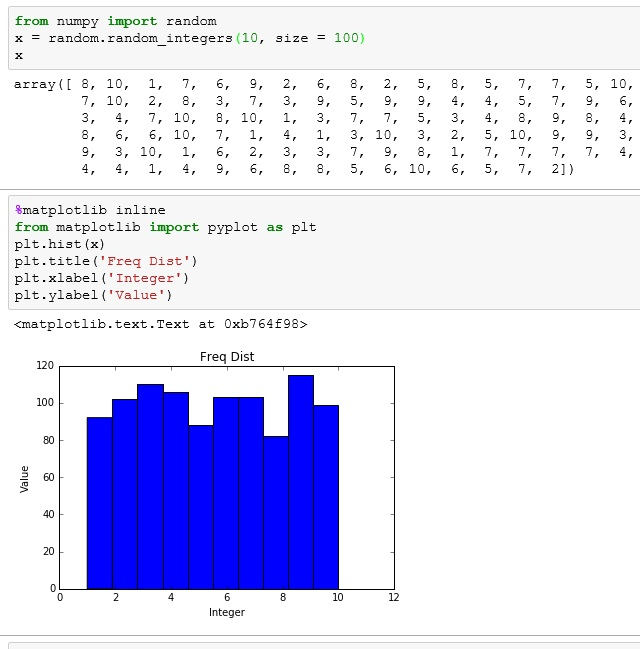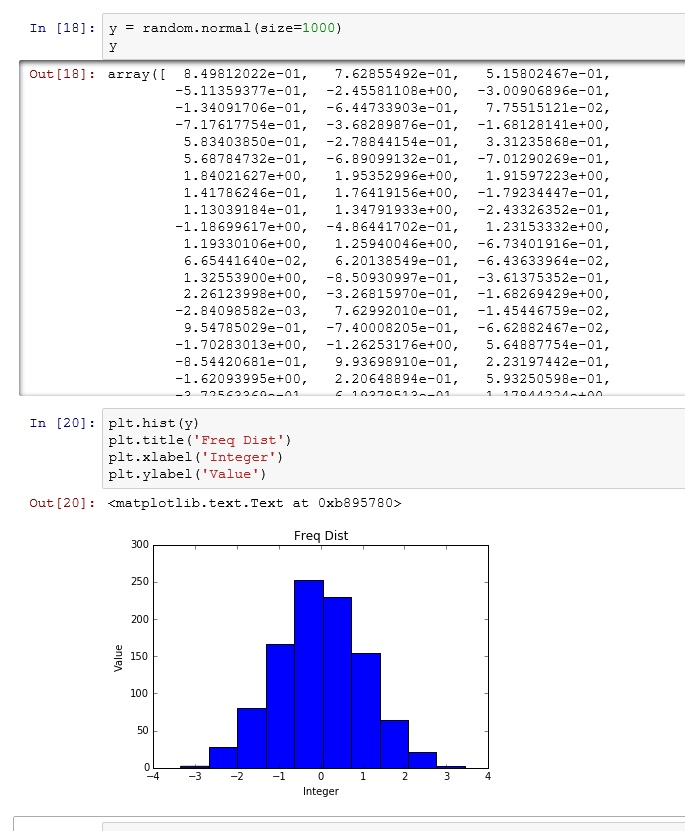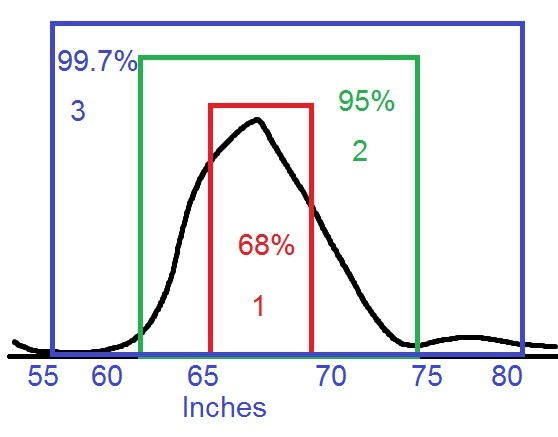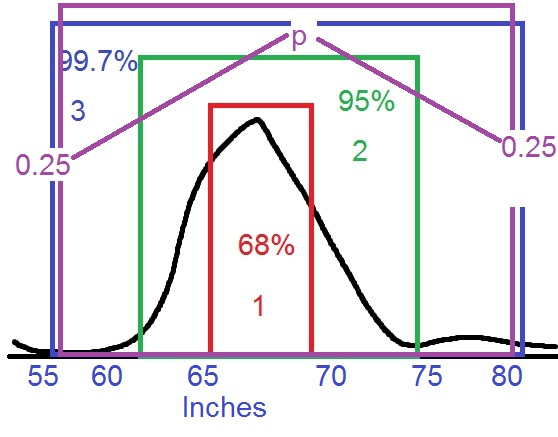# Python: Central Limit Theorem

The Central Limit Theorem is one of core principles of probability and statistics. So much so, that a good portion of inferential statistical testing is built around it. What the Central Limit Theorem states is that, given a data set – let’s say of 100 elements (See below) if I were to take a random sampling of 10 data points from this sample and take the average (arithmetic mean) of this sample and plot the result on a histogram, given enough samples my histogram would approach what is known as a normal bell curve.

In plain English

• Take a random sample from your data
• Take the average of your sample
• Plot your sample on a histogram
• Repeat 1000 times
• You will have what looks like a normal distribution bell curve when you are done.For those who don’t know what a normal distribution bell  curve looks like, here is an example. I created it using numpy’s normal methodIf you don’t believe me, or want to see a more graphical demonstration – here is a link to a simulation that helps a lot of people to grasp this concept: link

## Okay, I have bell curve, who cares?

The normal distribution of (Gaussian Distribution – named after the mathematician Carl Gauss) is an amazing statistical tool. This is the powerhouse behind inferential statistics.

The Central Limit Theorem tells me (under certain circumstances), no matter what my population distribution looks like, if I take enough means of sample sets, my sample distribution will approach a normal bell curve.

Once I have a normal bell curve, I now know something very powerful.

Known as the 68,95,99 rule, I know that 68% of my sample is going to be within one standard deviation of the mean. 95% will be within 2 standard deviations and 99.7% within 3.So let’s apply this to something tangible. Let’s say I took random sampling of heights for adult men in the United States. I may get something like this (warning, this data is completely made up – do not even cite this graph as anything but bad art work)But reading this graph, I can see that 68% of men are between 65 and 70 inches tall. While less than 0.15% of men are shorter than 55 inches or taller than 80 inches.

Now, there are plenty of resources online if you want to dig deeper into the math. However, if you just want to take my word for it and move forward, this is what you need to take away from this lesson:

## p value

As we move into statistical testing like Linear Regression, you will see that we are focus on a p value. And generally, we want to keep that p value under 0.5. The purple box below shows a p value of 0.5 – with 0.25 on either side of the curve. A finding with a p value that low basically states that there is only a 0.5% chance that the results of whatever test you are running are a result of random chance. In other words, your results are 99% repeatable and your test demonstrates statistical significance.## One thought on “Python: Central Limit Theorem”

1.Parthiban Rajendran

Suppose my data is 10000 gumballs, with about 60% yellow balls. How do I do sampling distribution in python also showing CLT convergence to normal distribution from Bernoulli? Kindly help. https://stackoverflow.com/questions/51724711/sample-distribution-simulation-not-resulting-in-normal